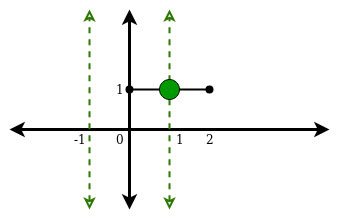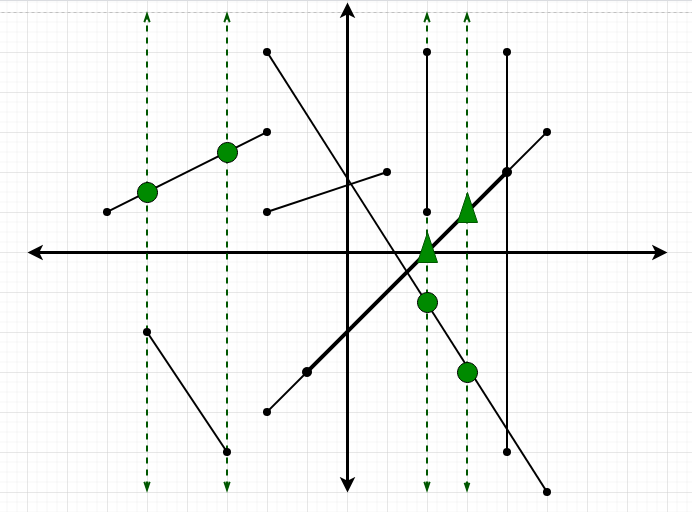# Count of intersections of M line segments with N vertical lines in XY plane

Given x coordinates of N vertical lines (parallel to Y-axis) and M line segments extending from (x1, y1) to (x2, y2), the task is to find the total number of intersections of the line segments with the vertical lines.

Examples:

Input: N = 2, M = 1, lines[] = {-1, 1}, Segments[] = {0, 1, 2, 1}
Output: 1
Explanation:
There is only one point of intersection (1, 1)Input: N = 4, M = 8, lines[] = {-5, -3, 2, 3}, segments[] = {{-2, 5, 5, -6}, {-5, -2, -3, -5}, {-2, 3, -6, 1}, {-1, -3, 4, 2}, { 2, 5, 2, 1}, { 4, 5, 4, -5}, {-2, -4, 5, 3}, { 1, 2, -2, 1}};
Output: 8
Explanation:
There are total of 8 intersections.
Dotted lines are the vertical lines.
A green circle denote a single point of intersection and
a green triangle denotes that two line segments
intersect same vertical line at that point.## Recommended: Please try your approach on {IDE} first, before moving on to the solution.

Naive Approach:
The simplest approach is, for each query, check if a vertical line falls between the x-coordinates of the two points. Thus, each segment will have O(N) computational complexity.
Time complexity: O(N * M)

Approach 2: The idea is to use Prefix Sum to solve this problem efficiently. Follow the steps below to solve the problem:

• The first observation we can make is that the y-coordinates do not matter. Also, we can observe that just touching the vertical line does not count as an intersection.
• First, compute a prefix array of the number of occurrences of vertical lines till now and then just subtract the number of occurrences till x2-1 (we don’t consider x2 as it just qualifies as touch and not as an intersection) from the number of occurrences till x1. So for each segment, computational complexity reduces to O(1).

Below is the implementation of the above approach.

## C++

 `// C++ implementation for the ` `// above approach. ` `#include ` `using` `namespace` `std; ` ` `  `// Function to create prefix sum array ` `void` `createPrefixArray(``int` `n, ``int` `arr[], ` `                       ``int` `prefSize, ` `                       ``int` `pref[]) ` `{ ` `    ``// Initialize the prefix array ` `    ``// to remove garbage values ` `    ``for` `(``int` `i = 0; i < prefSize; i++) { ` `        ``pref[i] = 0; ` `    ``} ` ` `  `    ``// Marking the occurences of ` `    ``// vertical lines ` `    ``for` `(``int` `i = 0; i < n; i++) { ` `        ``// x is the value after ` `        ``// Index mapping ` `        ``int` `x = arr[i] + 1000000; ` `        ``pref[x]++; ` `    ``} ` ` `  `    ``// Creating the prefix array ` `    ``for` `(``int` `i = 1; i < prefSize; i++) { ` `        ``pref[i] += pref[i - 1]; ` `    ``} ` `} ` ` `  `// Function returns the count of ` `// total intersection ` `int` `pointsOfIntersection(``int` `m, ` `                         ``int` `segments[], ` `                         ``int` `size, ` `                         ``int` `pref[]) ` `{ ` ` `  `    ``// ans is the number of points of ` `    ``// intersection of the line segments ` `    ``// with the vertical lines ` `    ``int` `ans = 0; ` ` `  `    ``for` `(``int` `i = 0; i < m; i++) { ` `        ``int` `x1 = segments[i]; ` `        ``int` `x2 = segments[i]; ` ` `  `        ``// Index mapping ` `        ``x1 = x1 + 1000000; ` `        ``x2 = x2 + 1000000; ` ` `  `        ``// We don't consider a vertical ` `        ``// line segment because even if ` `        ``// it falls on a verticale line ` `        ``// then it just touches it and ` `        ``// not intersects. ` `        ``if` `(x1 != x2) { ` `            ``// We have assumed that x1 ` `            ``// will be left and x2 right ` `            ``// but if not then we just ` `            ``// swap them ` `            ``if` `(x1 > x2) { ` `                ``swap(x1, x2); ` `            ``} ` ` `  `            ``int` `Occ_Till_Right = pref[x2 - 1]; ` `            ``int` `Occ_Till_Left = pref[x1]; ` ` `  `            ``ans = ans + (Occ_Till_Right ` `                         ``- Occ_Till_Left); ` `        ``} ` `    ``} ` `    ``return` `ans; ` `} ` ` `  `int` `main() ` `{ ` ` `  `    ``// N is the number of vertical lines ` `    ``// M is the number of line segments ` `    ``int` `N = 4; ` `    ``int` `M = 8; ` ` `  `    ``int` `size = 2000000 + 2; ` `    ``int` `pref[size]; ` ` `  `    ``int` `lines[N] = { -5, -3, 2, 3 }; ` ` `  `    ``// Format : x1, y1, x2, y1 ` `    ``int` `segments[M] = { { -2, 5, 5, -6 }, ` `                           ``{ -5, -2, -3, -5 }, ` `                           ``{ -2, 3, -6, 1 }, ` `                           ``{ -1, -3, 4, 2 }, ` `                           ``{ 2, 5, 2, 1 }, ` `                           ``{ 4, 5, 4, -5 }, ` `                           ``{ -2, -4, 5, 3 }, ` `                           ``{ 1, 2, -2, 1 } }; ` ` `  `    ``// First create the prefix array ` `    ``createPrefixArray(N, lines, size, pref); ` ` `  `    ``// Print the total number of intersections ` `    ``cout << pointsOfIntersection(M, segments, ` `                                 ``size, pref) ` `         ``<< endl; ` ` `  `    ``return` `0; ` `} `

## Python3

 `# Python3 implementation for the  ` `# above approach ` ` `  `# Function to create prefix sum array ` `def` `createPrefixArray(n, arr, prefSize, pref): ` ` `  `    ``# Initialize the prefix array  ` `    ``# to remove garbage values ` `    ``for` `i ``in` `range``(prefSize): ` `        ``pref[i] ``=` `0` ` `  `    ``# Marking the occurances ` `    ``# of vertical lines ` `    ``for` `i ``in` `range``(n): ` `        ``x ``=` `arr[i] ``+` `1000000` `        ``pref[x] ``+``=` `1` ` `  `    ``# Creating the prefix array ` `    ``for` `i ``in` `range``(``1``, prefSize): ` `        ``pref[i] ``+``=` `pref[i ``-` `1``] ` ` `  `# Function that returns the count  ` `# of total intersection ` `def` `pointOfIntersection(m, segments, size, pref): ` ` `  `    ``# ans is the number of points of  ` `    ``# intersection of the line segments ` `    ``# with the vertical lines ` `    ``ans ``=` `0` ` `  `    ``for` `i ``in` `range``(m): ` `        ``x1 ``=` `segments[i][``0``] ` `        ``x2 ``=` `segments[i][``2``] ` ` `  `        ``# Index mapping ` `        ``x1 ``=` `x1 ``+` `1000000` `        ``x2 ``=` `x2 ``+` `1000000` ` `  `        ``# we don't consider a vertical   ` `        ``# line segment because even if ` `        ``# it falls on a vertical line ` `        ``# then it just touches it and ` `        ``# not intersects. ` `        ``if` `(x1 !``=` `x2): ` `             `  `            ``# We have assumed that x1  ` `            ``# will be left and x2 right ` `            ``# but if not then just swap ` `            ``if` `(x1 > x2): ` `                ``x1, x2 ``=` `x2, x1 ` ` `  `            ``Occ_Till_Right ``=` `pref[x2 ``-` `1``] ` `            ``Occ_Till_Left ``=` `pref[x1] ` ` `  `            ``ans ``+``=` `(Occ_Till_Right ``-` `Occ_Till_Left) ` ` `  `    ``return` `ans ` ` `  `# Driver code ` ` `  `# Number of vertical lines ` `N ``=` `4`  ` `  `# Number of line segments ` `M ``=` `8`  ` `  `size ``=` `2000000` `+` `2` `pref ``=` `[``0``] ``*` `size  ` `lines ``=` `[ ``-``5``, ``-``3``, ``2``, ``3` `] ` ` `  `# Format : x1, y1, x2, y2 ` `segments ``=` `[ [ ``-``2``, ``5``, ``5``, ``-``6` `], ` `             ``[ ``-``5``, ``-``2``, ``-``3``, ``-``5` `], ` `             ``[ ``-``2``, ``3``, ``-``6``, ``1` `], ` `             ``[ ``-``1``, ``-``3``, ``4``, ``2` `], ` `             ``[ ``2``, ``5``, ``2``, ``1` `], ` `             ``[ ``4``, ``5``, ``4``, ``-``5` `], ` `             ``[ ``-``2``, ``-``4``, ``5``, ``3` `], ` `             ``[ ``1``, ``2``, ``-``2``, ``1` `] ] ` ` `  `# First create the prefix array ` `createPrefixArray(N, lines, size, pref) ` ` `  `# Print the total number of intersections ` `print``(pointOfIntersection(M, segments, size, pref)) ` ` `  `# This code is contributed by himanshu77 `

Output:

```8
```

Approach 3: To optimize the above approach, we can adopt a space efficient method using Map Data structure. Follow the steps below to solve the problem:

• We can make a map which stores the number of occurrences till now, just with the difference from the first approach is that we insert only the co-ordinates that have the vertical lines.
• When we want to search the number of intersections from x1 to x2, we can just subtract lower_bound(x1+1) from upper_bound(x2-1) of the map.

Below is the implementation of the above approach.

## C++

 `// C++ implementation for the ` `// above approach. ` `#include ` `using` `namespace` `std; ` ` `  `// Function to create map that stores ` `// the number of occurences of the ` `// vertical lines till now. ` `map<``int``, ``int``> createMap(``int` `n, ` `                        ``int` `arr[]) ` `{ ` `    ``map<``int``, ``int``> mp; ` ` `  `    ``sort(arr, arr + n); ` `    ``for` `(``int` `i = 0; i < n; i++) { ` `        ``mp.insert({ arr[i], i + 1 }); ` `    ``} ` `    ``return` `mp; ` `} ` ` `  `// Function returns the count of ` `// total intersections ` `int` `pointsOfIntersection(``int` `m, ` `                         ``int` `segments[], ` `                         ``int` `n, ` `                         ``map<``int``, ``int``> pref) ` `{ ` `    ``// ans stores the number ` `    ``// of intersections ` `    ``int` `ans = 0; ` ` `  `    ``// Loop over all the segments ` `    ``for` `(``int` `i = 0; i < m; i++) { ` `        ``int` `x1 = segments[i]; ` `        ``int` `x2 = segments[i]; ` `        ``if` `(x1 == x2) { ` `            ``continue``; ` `        ``} ` ` `  `        ``// For convenience we make x1 as ` `        ``// x co-ordinate of left point ` `        ``// and x2 as x co-ordinate of ` `        ``// right point. ` `        ``if` `(x1 > x2) { ` `            ``swap(x1, x2); ` `        ``} ` ` `  `        ``auto` `it1 = *pref.lower_bound(x1 + 1); ` `        ``auto` `it2 = *pref.upper_bound(x2 - 1); ` ` `  `        ``int` `intersections = 0; ` ` `  `        ``// If x co-ordinate of the left point ` `        ``// is after the last vertical line ` `        ``// then we dont add anything. ` `        ``if` `(pref.lower_bound(x1 + 1) ` `            ``== pref.end()) { ` `            ``intersections = 0; ` `        ``} ` `        ``// If there is no occurence of any ` `        ``// vertical line after (x2-1) ` `        ``// then we can mark the ` `        ``// number of intersections as ` `        ``// n - occurrences till x1 ` `        ``// because the total occurrences ` `        ``// are n and all of them ` `        ``// will fall before x2. ` `        ``else` `if` `(pref.upper_bound(x2 - 1) ` `                 ``== pref.end()) { ` `            ``intersections ` `                ``= n - it1.second + 1; ` `        ``} ` `        ``else` `{ ` `            ``intersections ` `                ``= it2.second ` `                  ``- it1.second; ` `        ``} ` `        ``ans += intersections; ` `    ``} ` `    ``return` `ans; ` `} ` ` `  `// Driver code ` `int` `main() ` `{ ` `    ``// N is the number of vertical lines ` `    ``// M is the number of line segments ` `    ``int` `N = 4; ` `    ``int` `M = 8; ` ` `  `    ``int` `lines[N] = { -5, -3, 2, 3 }; ` ` `  `    ``// Format : x1, y1, x2, y1 ` `    ``int` `segments[M] ` `        ``= { { -2, 5, 5, -6 }, ` `            ``{ -5, -2, -3, -5 }, ` `            ``{ -2, 3, -6, 1 }, ` `            ``{ -1, -3, 4, 2 }, ` `            ``{ 2, 5, 2, 1 }, ` `            ``{ 4, 5, 4, -5 }, ` `            ``{ -2, -4, 5, 3 }, ` `            ``{ 1, 2, -2, 1 } }; ` ` `  `    ``map<``int``, ``int``> pref = createMap(N, lines); ` ` `  `    ``cout << pointsOfIntersection( ` `                ``M, ` `                ``segments, ` `                ``N, pref) ` `         ``<< endl; ` `    ``return` `0; ` `} `

Output:

```8
```My Personal Notes arrow_drop_upCheck out this Author's contributed articles.

If you like GeeksforGeeks and would like to contribute, you can also write an article using contribute.geeksforgeeks.org or mail your article to contribute@geeksforgeeks.org. See your article appearing on the GeeksforGeeks main page and help other Geeks.

Please Improve this article if you find anything incorrect by clicking on the "Improve Article" button below.

Improved By : himanshu77